# Why this simple one transistor amp doesn't work?

#### babaliaris

Joined Nov 19, 2019
158As you can see the current in Rout and Vout are zero.

The following is without the Cout capacitor: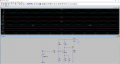1) Why is the I(Rout) current constant? Shouldn't it be ac? I only found one way to explain this:
a) The ac starts only in the collector's path (after vout, Rc node).
b) The Iac(R1) will not split because Vcc is shorted in AC analysis so Iac(R1) will fully go into the ground.
c) This means that I(Rc) will be fully DC.
d) Which means that when Idc(Rc) splits, one current will be the I(Rout) = 26mA and the other will lift Ic up.

The emitter's current is also dc is this normal?

1 is also explaining why I(Rout) and Vout are zero in the first image. It's because there is only dc there so the capacitor
blocks it.

So what am I missing? Am I taking the wrong node as output?

Thank you!

#### Attachments

• 1.5 KB Views: 2

#### ericgibbs

Joined Jan 29, 2010
16,741
hi baba,
E

Update:
Just about everything on that circuit is wrong.Last edited:
•DickCappels and babaliaris

#### Ian0

Joined Aug 7, 2020
6,645
Your input capacitor (1uF) has an impedance of 159kΩ at 1Hz.
your input signal is attenuated by the capacitor and the input resistance (83Ω), so there will be only 2.6mV going into the amplifier, which might have a gain of about 5. So the output is likely to be <13mV, which is then attenuated by the 159kΩ impedance of the output capacitor and the 100Ω load, giving an output of about 8uV.

Make the capacitors much larger, make the input resistance 100 times larger and test at 1kHz.

•babaliaris

#### babaliaris

Joined Nov 19, 2019
158
I'm getting what you are saying. I thought capacitance doesn't affect the amplifier but only the range of frequencies you can input and output.

I probably need to see the math behind this to fully understand it, which I'm currently working on it.

Just to keep you noted, I'm currently learning how to do AC analysis on the equivalent transistor model (Dependent current source with a bunch of resistors) and how to calculate the gain (vout/vin).

#### Ian0

Joined Aug 7, 2020
6,645
I'm getting what you are saying. I thought capacitance doesn't affect the amplifier but only the range of frequencies you can input and output.
True, but 1uF and 83Ω on the input puts the Lower end of that range at 1.9kHz, and you are testing at 1Hz.

•babaliaris

#### LvW

Joined Jun 13, 2013
1,586
I'm currently learning how to do AC analysis on the equivalent transistor model (Dependent current source with a bunch of resistors) and how to calculate the gain (vout/vin).
At first, I recommend urgently to study the various steps for designing (and understanding) the working principles for such a gain stage.
How did you arrive at the resistor values? Normally, one starts with the desired collector current. And as a second step the resistor values are calculated for meeting this value.
Your resistor values are not "optimal" (too low).

•babaliaris

#### babaliaris

Joined Nov 19, 2019
158
How did you arrive at the resistor values?
Your resistor values are not "optimal" (too low).

Normally, one starts with the desired collector current. And as a second step, the resistor values are calculated for meeting this value.
Can you give me a hint on how to do that? I've already thought of it but still, I can't calculate the rest of the values because I don't know the transistor voltages plus the missing resistances.

For example: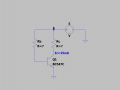Even if I knew Hfe (people told me to not trust this value. Even by experimenting, when I measure Ib, Ic in my circuits and then calculate Ic/Ib it never gives me the same value so how the f Hfe is a constant?)
and knew Ib = Ic/Hfe, Ie = (Hfe + 1)Ib I'm stil missing a lot of stuff.

If I do KVL@(Ground -> Source -> Rc -> Ground) I don't know both Rc and Vce.
$I_{c} \cdot R_{c} + V_{ce} = 5$

If I do KVL@(A -> Rb -> base -> Rc -> A) I don't know Rb, Rc and Vbc
$I_{b} \cdot R_{b} - V_{bc} - I_{c} \cdot R_{c} = 0$

If I know at least one of Vbe, Vcb, Vce can I calculate everything?

Can you show me how you would approach that?

Thank you!!!

#### Attachments

• 571 bytes Views: 2
Last edited:

#### ericgibbs

Joined Jan 29, 2010
16,741
hi baba,
E

•babaliaris

#### Ian0

Joined Aug 7, 2020
6,645
Start by deciding on the collector voltage. Choose half supply, so that you can get the biggest possible output signal.
Decide on the collector current - you have a free choice, but it depends on what you wish to drive from the output. If it’s just a signal output, it will generally have to drive about 10k, or about 100uA from a 1 Volt signal. So ten times the output signal is a good place to start, so that the output doesn‘t put too much load on the amplifier.
Then you can calculate the collector resistor.
You need a feedback network to set the output DC conditions. The current in the feedback network should be about times the base current, and the base current will be collector current divided by hfe.
Use a resistor from output to base, and a resistor from base to ground. Assume Vbe is about 0.6V.
You can then calculate all the resistors.

•anhnha, absf and babaliaris

#### dl324

Joined Mar 30, 2015
15,405
So what am I missing?
You're using a circuit simulator and you can't figure out why your circuit isn't working??

You're using a 5.25V supply, but you're trying to input a 10V p-p signal and you've biased the base to 0.875V. How is that supposed to work?

#### Papabravo

Joined Feb 24, 2006
19,551
Let me understand your proposition. You assigned the component values randomly and you have the unmitigated gall to ask why it isn't working. I should think that answer would be obvious even to the "noobiest" of members here. If that was a truly effective strategy then there would be no point in getting an engineering education at large cost in time and treasure, and you could make unlimited amounts of money by assigning component values randomly. Glad I don't live in that universe.

#### Papabravo

Joined Feb 24, 2006
19,551
You're using a circuit simulator and you can't figure out why your circuit isn't working??

You're using a 5.25V supply, but you're trying to input a 10V p-p signal and you've biased the base to 0.875V. How is that supposed to work?
I suppose any random answer will suffice.

#### crutschow

Joined Mar 14, 2008
31,089
I hate to break it to you, but random selection of electronic part values seldom work.Garbage in, garbage out.

#### dl324

Joined Mar 30, 2015
15,405
Literally, you're abusing the generosity of members who actually want to help other members who actually want to learn.

#### Papabravo

Joined Feb 24, 2006
19,551
Outrageously entitled behavior. No excuse for it.

#### babaliaris

Joined Nov 19, 2019
158
I don't understand the hate. Of course, when you do electronics you don't put things randomly, but now I don't understand everything and I just play with LTspice to see the behavior using simulations and try to understand what is going on plus studying the math behind them.

This is why I asked this question, in an attempt that some answers might give me some push about which parts are wrong
and how to fix it. It won't make me a master in designing transistor circuits but it might give some idea of what is going on.

And @Ian0 did that and I thank him for that. I'm really close to understanding how to choose the correct voltage, Ic values to calculate
the resistances I need in order to make my circuit behave as I please.

I never meant to insult anyone.

#### ericgibbs

Joined Jan 29, 2010
16,741
hi baba,
Please ignore the bad manners of your fellow members.Let's go through your circuit design.
E

•babaliaris

#### Ian0

Joined Aug 7, 2020
6,645
I think some of you are being a little harsh. In most circuits there is a component the value of which is an arbitrary choice, so what value do you choose? Only experience tells you, and even then, once in a while it tells you wrong.

•babaliaris

#### ericgibbs

Joined Jan 29, 2010
16,741
hi baba,
In your last asc circuit you show 15mA collector current, let's assume a Vsupply of say 20Vdc, and you want the collector voltage to be Vsupply/2

What will the value of Rc resistor have to be.??

E

#### babaliaris

Joined Nov 19, 2019
158
Start by deciding on the collector voltage. Choose half supply, so that you can get the biggest possible output signal.
Decide on the collector current - you have a free choice, but it depends on what you wish to drive from the output. If it’s just a signal output, it will generally have to drive about 10k, or about 100uA from a 1 Volt signal. So ten times the output signal is a good place to start, so that the output doesn‘t put too much load on the amplifier.
Then you can calculate the collector resistor.
You need a feedback network to set the output DC conditions. The current in the feedback network should be about times the base current, and the base current will be collector current divided by hfe.
Use a resistor from output to base, and a resistor from base to ground. Assume Vbe is about 0.6V.
You can then calculate all the resistors.
Thank you for trying to make me understand!

I still have some questions though. In order to make you understand what is going on in my head, I will compare how I understand and solve the same problem with a diode: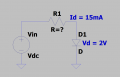$I_{d} \cdot R + V_{d} = V_{in} <=> R = \frac{Vin-V_{d}}{I_{d}}$ (1)

I know Vin, and with a correct (Id,Vd) = (15mA, 2V) point I can calculate the resistance that I need.

The catch here is to figure out which range of voltages you can use as the domain of equation (1) in order to
get an answer that is possible according to the characteristic I,V of the diode.For example, in the above picture, let's say my diode is a Red LED. In the graph, I can see that approximately
if I want my diode to allow 15mA, I need about Vd >= 1.5V . So basically I can say that for Id=15mA:

$R = \frac{Vin-V_{d}}{I_{d}} , \forall V_{d} \in (1.5, \infty)$ (1)

Now back to transistors. I don't know how to do that. In a diode, you need 2 pieces of information chosen so that they can "happen". So basically you need 2 values.

In a transistor how many do you need? Is it 4? (Ic, Vbe, Vce, Hfe)? After choosing your Ic current how do you properly choose (Vbe, Vce) so that this can actually happen? The datasheets have a lot of plots which I don't understand.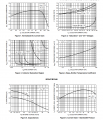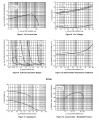I really don't know which graph to use and compared to a diode they seem weird and hard to understand.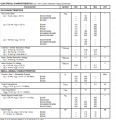Which one do I use? The datasheet is telling me that for different Ic I have different Hfe? I thought Hfe was dependent on temperature. Is it because when Ic becomes large, the transistor heats up a lot so Hfe changes?
Then how do I use it in my calculations if it's not trustworthy?

If I understand all of these, I think I will make great progress!